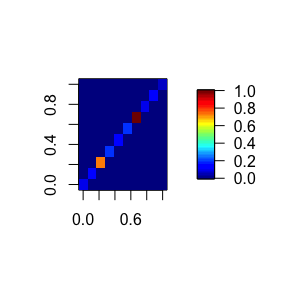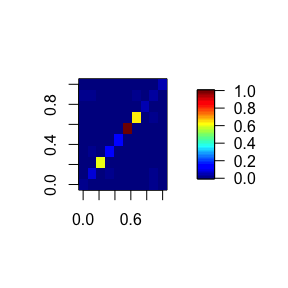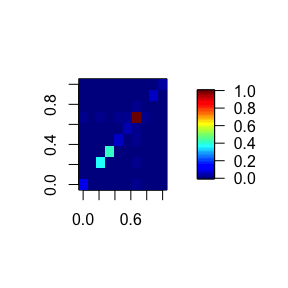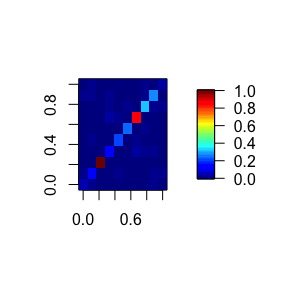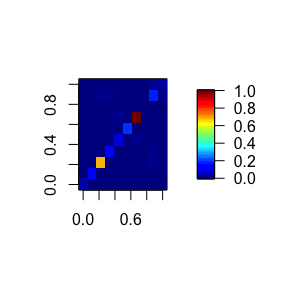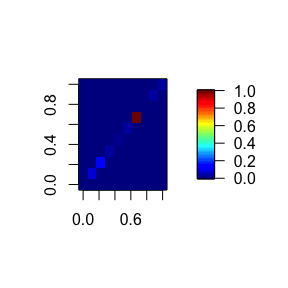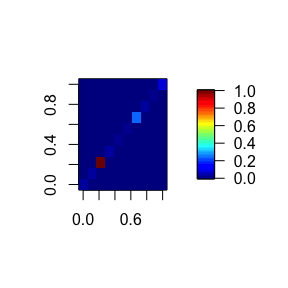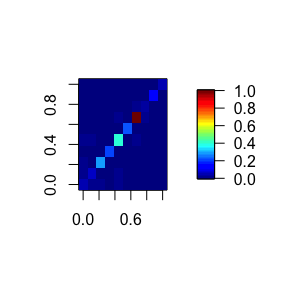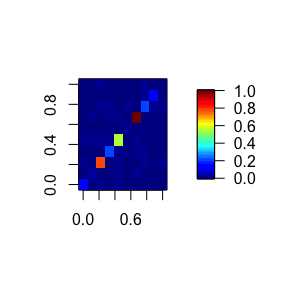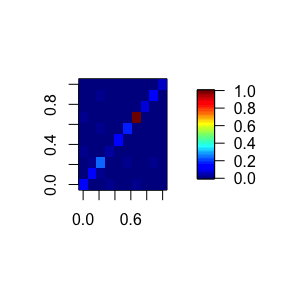# Learning cyclic causal graphs with backShift

#### 2017-09-07

This is an example for how to run backShift, an algorithm to estimate the connectivity matrix of a directed (possibly cyclic) graph with hidden variables. The underlying system is required to be linear and we assume that observations under different shift interventions are available.

In this example, we simulate a data set with the function simulateInterventions() provided in this package.

## Options

### Preliminaries

First, we load the backShift package as well as the packages ggplot2 and fields which are needed to visualize the results. Additionally, we set the random seed for reproducability.

# load package
require(backShift)
require(ggplot2)
require(fields)

# set seed
seed <- 1
set.seed(seed)

### Algorithm

backShift exploits differences between covariance or Gram matrices. To use covariance matrices set useCov to TRUE.

# use covariance matrix instead of Gram matrix
useCov <- TRUE 

### Stability selection

backShift estimates the connectivity matrix of a directed (possibly cyclic) graph. In order to control the number of falsly selected edges we can use stability selection which provides a finite sample control for the expected number of false discoveries. If stability selection should not be used, set the parameter EV to 0.

# bound on expected number of false selections for stability selection
EV <- 2
# selection threshold for stability selection
thres <- 0.75 

To visualize the point estimate of the connectivity matrix, the coefficients are thresholded at the absolute value given by thres.pe. So edges with coefficients smaller than thres.pe in absolute value are not displayed.

# threshold for point estimate
thres.pe <- 0.25

### Simulation

A data set can be simulated with simulateInterventions(). This function takes the true connectivity matrix as an input. A connectivity matrix can be generated with generateA() or you can use the connectivity matrix provided through data("exampleAdjacencyMatrix").

#### Connectivity matrix

In the following code, we set whether we “provide” the adjacency matrix A – e.g. by loading the example with data("exampleAdjacencyMatrix") or by creating an adjacency matrix ourselves – or whether we generate the connectivity matrix A with generateA().

NOTE: The entry A{ij} contains the edge from node i to node j.

# number of variables
p <- 10

# set whether to provide or to generate the adjacency matrix A
providedA <- TRUE

if(providedA){
p <- 10
}else{
# parameters to generate A

# should A be cyclic?
cyclic <- TRUE
# expected number of neighbors per node
expNumNeigh <- 0.1*p
# range for coefficients
minCoef <- 0.3
maxCoef <- 0.8

## Generate A -------
cat("Generating A...\n")
A.gen.result <- generateA(p, expNumNeigh, minCoef, maxCoef, cyclic)
A <- A.gen.result$A cat("A has a cycle of size", A.gen.result$sizeCycle, "\n")
}

#### Observations under different shift interventions

The following code generates a data set. In addition to the size of the data set, we can specify the following options:

• whether to also simulate observational data (simulateObs),
• whether hidden variables should be present (hidden)
• whether the location of the interventions should be known (knownInterventions; note that this is not needed for backShift)
• if knownInterventions is TRUE, fracVarInt gives the fraction of variables that are intervened on in each environment
• intMult determines the magnitude of the intervention variances (please see the manuscript for more details)
• noiseMult determines the noise variance
• nonGauss specifies whether to generate non-Gaussian noise
# number of observations
n <- 10000
# number of environments
G <- 10
# also simulate observational data?
simulateObs <- TRUE
# should hidden vars be included?
hidden <- FALSE
# should the location of the interventions be known?
knownInterventions <- FALSE
# if the location of the interventions is known, how many vars. should
# be intervened on in each environment (as a fraction of p)
fracVarInt <- 0.5
# multiplier for interventions (m_I in manuscript)
intMult <- 1.5
# multiplier for interventions (m_e in manuscript)
noiseMult <- 1
# simulate non-Gaussian noise?
nonGauss <- FALSE

## Simulate data -------
cat("Simulating the data...\n")
simulation.res <- simulateInterventions(n, p, A, G, intMult, noiseMult,
nonGauss, hidden, knownInterventions,
fracVarInt, simulateObs, seed)

# extract X, environment vector and index of observational data
X <- simulation.res$X env <- simulation.res$environment
baseInd <- simulation.res$configs$indexObservationalData

## Running backShift

We can now run backShift. Since we also generated observational data, we provide the corresponding index as baseInd. This is useful for estimating the intervention variances (see below).

## Run backShift -------
backshift.res <- backShift(X, env, covariance=useCov, ev=EV, threshold=thres,
baseSettingEnv = baseInd, tolerance = 1e-6,
verbose = FALSE)
## backShift: Percentage of runs in stability selection that converged: 100%

## Results

### Estimated graphs

Plot true graph:

# extract estimates
Ahat <- backshift.res$Ahat Ahat.structure <- backshift.res$AhatAdjacency

# compute performance metrics and plot result
cat("Plotting true graph... \n")
plotGraphEdgeAttr(estimate = A, plotStabSelec = FALSE, labels = colnames(A),
thres.point = 0, thres.stab = thres, main = "True graph")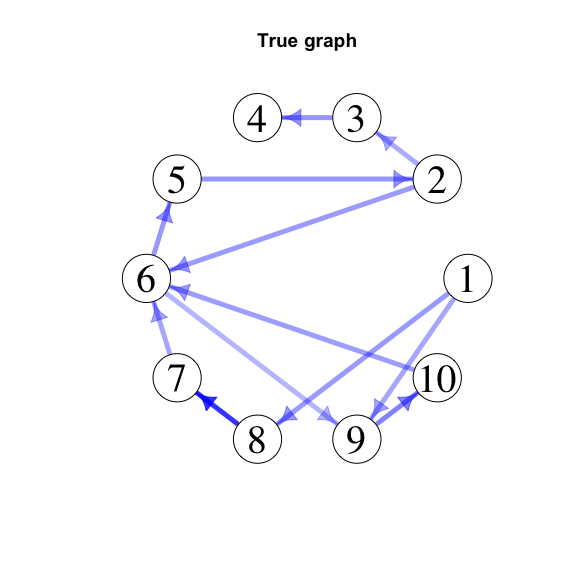Plot point estimate (thresholded at thres.pe): The edge intensity reflects the relative magnitude of the coefficients.

cat("Plotting point estimate, thresholded at", thres.pe,"... \n")
plotGraphEdgeAttr(estimate = Ahat, plotStabSelec = FALSE, labels = colnames(A),
thres.point = thres.pe, thres.stab = thres,
main = paste("Point estimate thresholded at", thres.pe))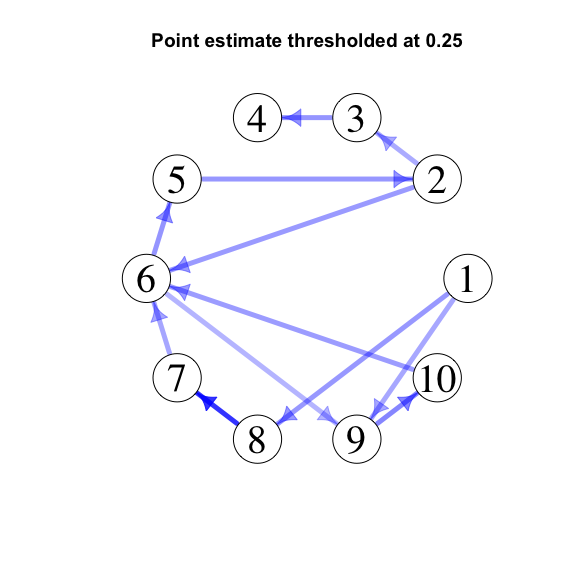Plot stability selection result: The edges thickness indicates how often an edge was selected in the stability selection procedure.

cat("Plotting stability selection result... \n")
plotGraphEdgeAttr(estimate = Ahat.structure, plotStabSelec = TRUE,
labels = colnames(A), thres.point = thres.pe,
edgeWeights = Ahat, thres.stab = thres,
main = "Stability selection result")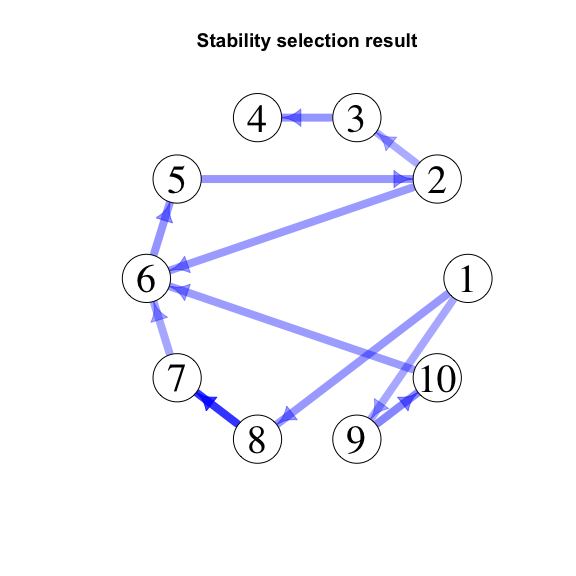### Metrics

metricsThreshold computes the structural hamming distance (SHD), true positive rate (TPR)/recall, false positive rate (FPR) and precision. The connectivity matrix gets thresholded at thres prior to computing these metrics.

# metrics for point estimate, thresholded at thres.pe
metricsThresholdedA <- metricsThreshold(A, Ahat, thres = thres.pe)

# metrics for stability selection result
metricsStabSelection <- metricsThreshold(A, Ahat.structure, thres = 0)
Metrics for point estimate, thresholded at 0.25
Threshold SHD TPR/Recall FPR Precision
0.25 0 1 0 1
Metrics for stability selection result
Threshold SHD TPR/Recall FPR Precision
0 1 0.9167 0 1

## Estimating the intervention variances

The location and the strength of the shift interventions can be estimated from the data (up to an offset if no observational data is present). These estimates are returned by the function backShift() as a G x p- dimensional matrix varianceEnv where G is the number of environments and p is the number of variables. The ij-th entry contains the difference between the estimated intervention variance of variable j in environment i and the estimated intervention variance of variable j in the base setting (given by input parameter baseSettingEnv).

plotInterventionVars(backshift.res$varianceEnv, simulation.res$interventionVar)
## Warning: Ignoring unknown aesthetics: type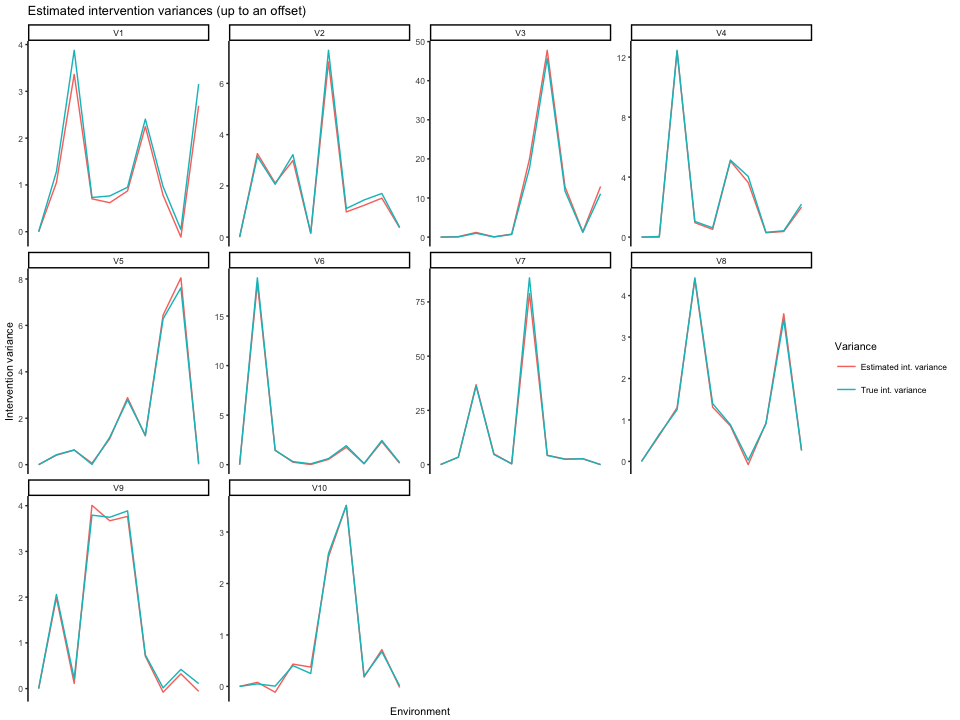## Checking the model assumptions

We can check the model assumptions to some extent by the success or failure of the joint diagonalization procedure. The plots below show that the joint diagonalization succeeded for all environments as all matrices are diagonal. The values are scaled to [0,1] to allow for comparability accross plots.

for(i in 1:G){
plotDiagonalization(estConnectivity = backshift.res\$Ahat, X = X, env = env, whichEnv = i)
}